## Making excellent histograms on your calculator

Your graphing calculator will make superb histograms from data you enter. If you have tried to do this, but your histograms don’t look the way you expect, follow this procedure. You have to tweak the x-min, x-max, and x-scale values to match your preferences. Let’s say that you have collected the following data (perhaps they are the heights of 30 people in your class):

61.0    61.5     62.0    65.0    65.5     65.5     66.0     66.0
66.5    66.5     67.0    67.0    67.5     68.0     68.5     68.5
69.0    69.0    69.0    69.5    69.5     70.0     70.5     72.0
72.0    72.0    74.0    74.0    75.0     75.5

 1. Determine the smallest class value and the class width. For this data set, I would display the data in five classes. We can set the smallest class value to 61, the largest class value to 76 and therefore the class width will be 3. 2. On your calculator, enter the data into a list. Here I’ve put it into L1.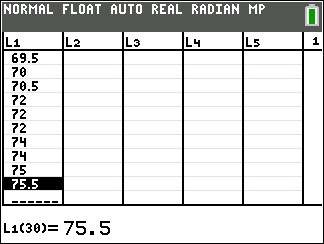3. Go to STAT PLOT and turn one of the plots on. 4. Select the histogram type and if necessary specify the Xlist location.5. Press zoom and select 9 (ZoomStat). Your graph probably won’t look right, but we’ll fix that. 6. Press the WINDOW button and set Xmin= to the smallest class value, Xmax= to the largest class value, and Xscl= to the class width. You might also need to adjust the Ymin and Ymax values.7. Press GRAPH, and admire your excellent histogram.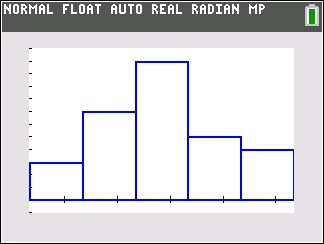## graphing sec x, csc x functions

Graphing secant functions and cosecant functions can be daunting for students. But you can tame them if you recognize how they are related to graphs of sine and cosine functions. If you can graph the associated sine or cosine function, then secant and cosecant graphs will be pretty easy for you.

We will demonstrate this process with an example: Draw the graph of$-3 \csc{(2x+\dfrac{\pi}{2})} - 1$

The first step for you is to graph a different function. If you are asked to graph a cosecant curve as in this example, change csc to sin and graph that instead. If you are graphing a secant function, change sec to cos and graph that instead. In our example, we will graph$-3 \sin{(2x+\dfrac{\pi}{2})} - 1$

I showed you how to graph sine and cosine functions in a previous post, so check that out if you need help. In that post, we graphed the sine function listed above. Here, we will assume you have already graphed the sine function: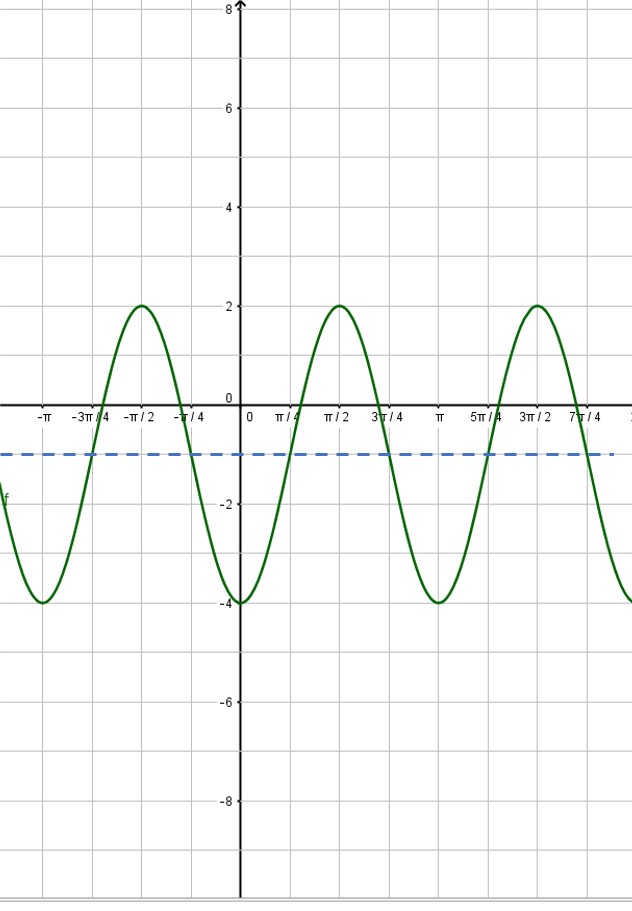Next, put in the vertical asymptotes everywhere the sine curve crosses the midline: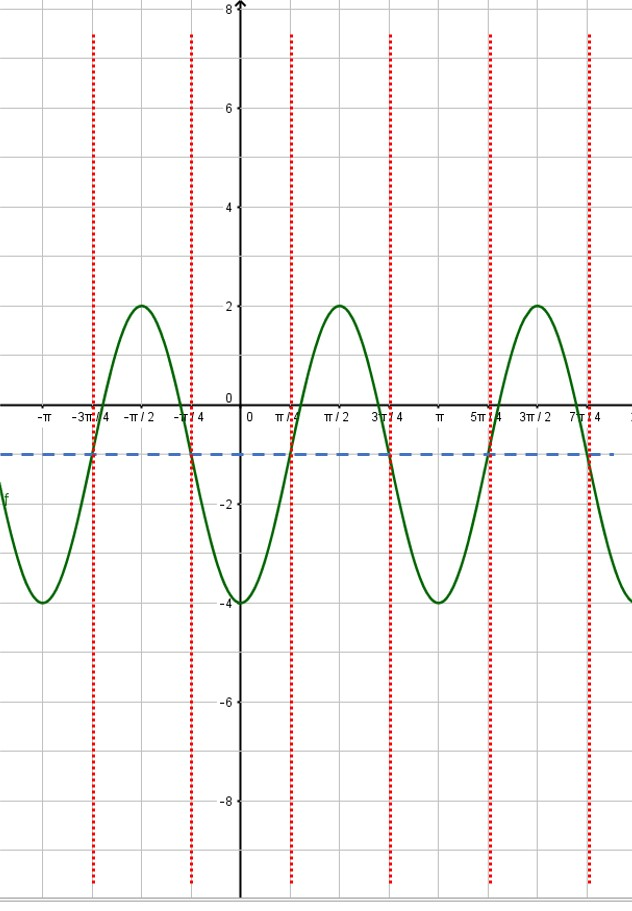Then use the asymptotes to place the branches of the cosecant function. Also, notice that the minimum points of the cosecant curve touch the maximum points of the sine curve and vice versa: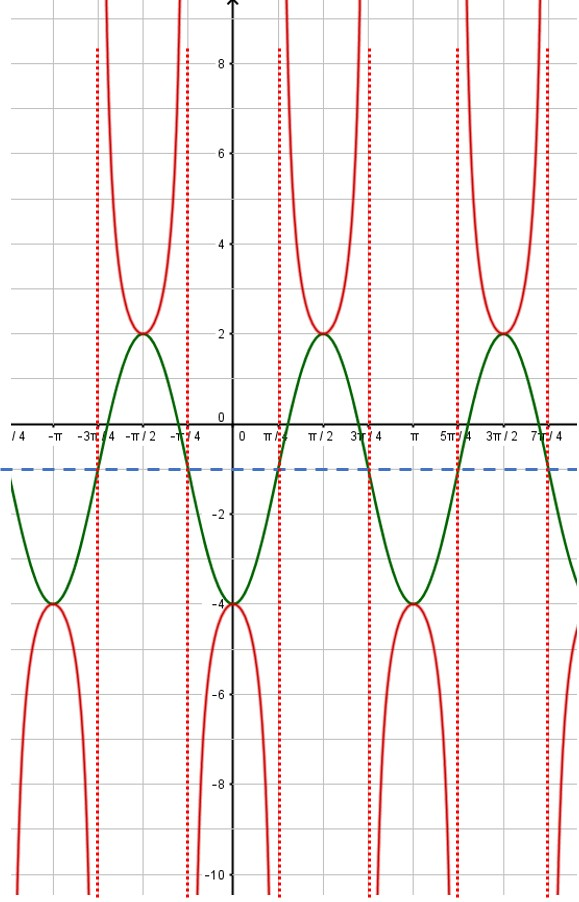That’s all there is to it! You can erase the sine curve (in green) or you can leave it. I usually draw in the sine or cosine curve in light pencil or as a dashed line, so that it is obvious which curve is the secant or cosecant curve. Here, the red curve is the graph we desire.

## Graphing sine and cosine functions like a pro

When graphing a sine or cosine curve, the first thing you must do is determine the amplitude, period, phase shift and vertical shift. See my previous post (Graphing Sine and Cosine Functions – Intro) if you need help with this analysis. In this post, we will graph the function$\displaystyle f(x) = -3 \sin (2x + \frac{\pi}{2}) -1$

We quickly determine the four values we need:

Amplitude = |-3| = 3

Period =$2 \pi /2 = \pi$

Phase shift =$-(\pi /2)/2 = - \pi/4$ (that is,$\pi /4$ units to the left)

Vertical shift = -1

This is all the information we need in order to complete the graph. Just follow this procedure step-by-step.

 1. Put values on the coordinate axes. On the y-axis, you typically make each square equal to one unit, but you can change this if you want. To determine the scale on the x-axis, take the period and divide by 4. This will be the scale on the x-axis. In our example, the period is$\pi$, so each square will be$\pi /4$. The vertical axis will be one unit per square. What do you do if your teacher gives you a grid with the numbers already in place? You should get a blank piece of graph paper and do your own grid!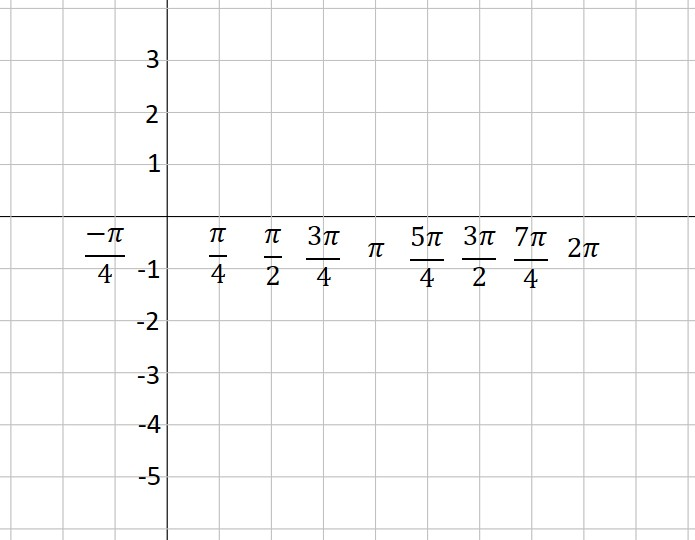2. Use the vertical shift to draw a dashed line across the figure. This is the location of the midline of your graph. In our example, the vertical shift is -1, so we draw a dashed line at y= -1.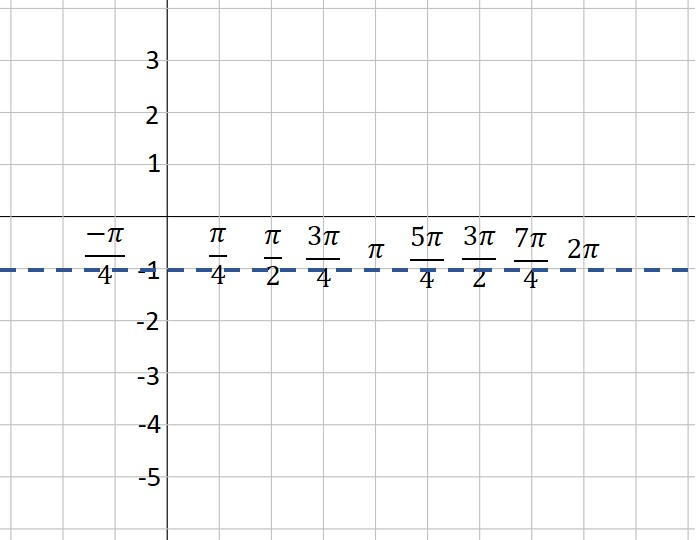3. Use the amplitude to draw two more dashed lines—one above the midline and one below. These represent the maximum and minimum values of your function. In our example, the amplitude is 3. Three units above -1 is 2—that’s our maximum dashed line. Three units below -1 is -4—that’s where our minimum is located.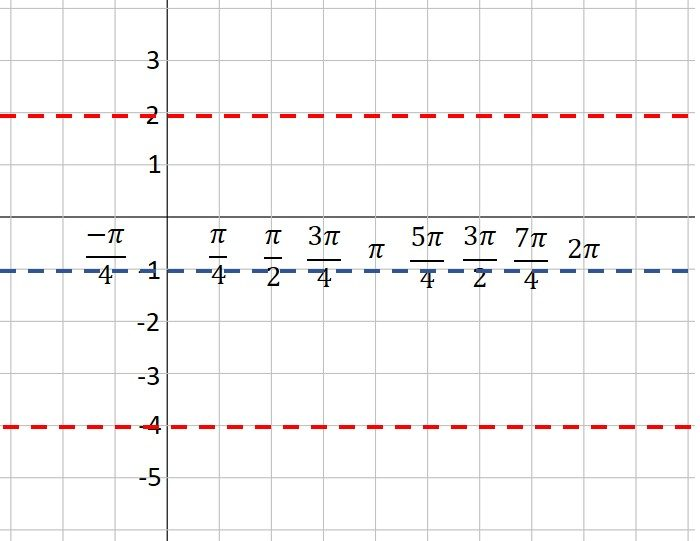4. Plot the starting point of your graph, using the vertical shift and phase shift as a guide. Our function is a sine curve, which starts at the midline. The phase shift is$\pi /4$ to the left, so our initial point is$\pi /4$ units left of the y-axis. If our function had been a cosine curve, our initial point would be plotted on the maximum line instead of the midline (or on the minimum line if A is negative). It’s hard to see, but note that I’ve placed a green dot at the “start” point; the coordinates are$( - \pi /4, -1).$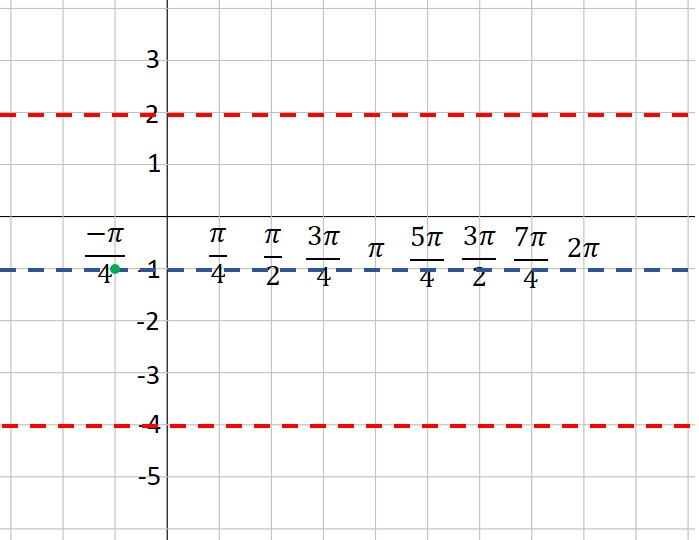5. Moving one square to the right at a time (because each square is one quarter of a period), plot points at the maximum, midline, minimum and midline. This is one period of your function. If you want to graph more than one period, continue the process. In our example, we’ve plotted points for two complete periods. Note that because A is a negative number (-3), our first point after the starting point is at the minimum instead of the maximum. Look closely, and you will see that I’ve placed a green dot every square to the right of our first point.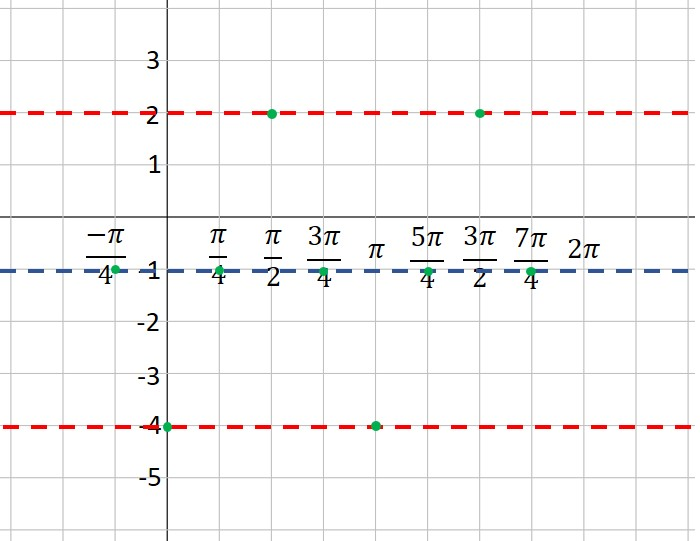6. Connect the dots with a nice smooth curve. You’ve graphed the sine curve like a pro!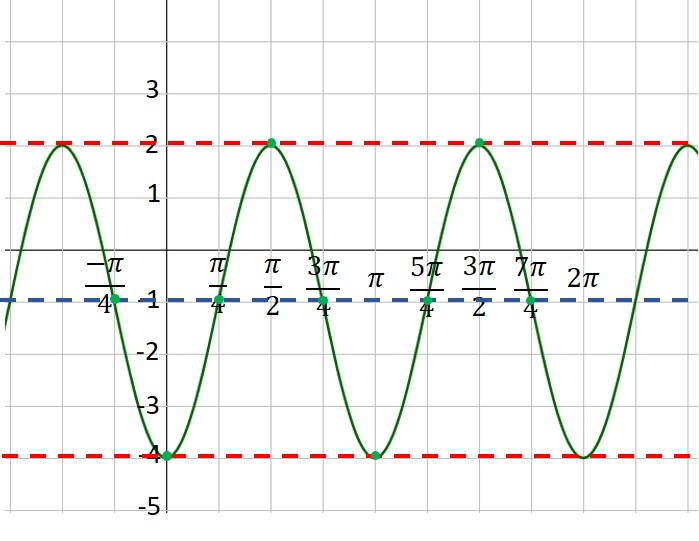## Graphing sine and cosine functions– an Intro

One of the most complicated skills you need to learn in your trig class is how to graph sine and cosine functions. This scares a lot of students, but you can tame this process if you make one simple observation: Every sine and cosine curve has exactly the same shape! No matter the amplitude or period or phase shift, the curve looks just like this: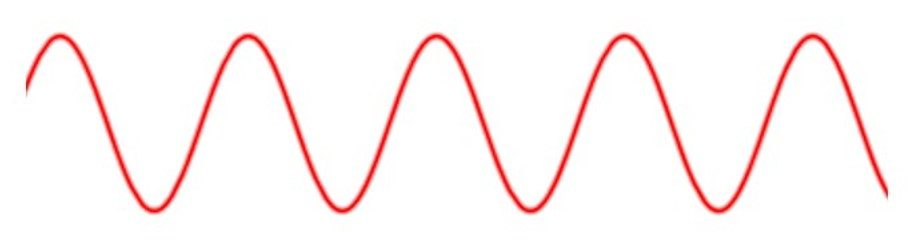You only need to place the graph in its proper position on the coordinate axes. This is (mostly) easy to accomplish if you can remember only two things about the sine and cosine parent curves:

1)     The sine curve y = sin x “starts” at the origin and goes up to its maximum, while the cosine curve y = cos x “starts” at its maximum.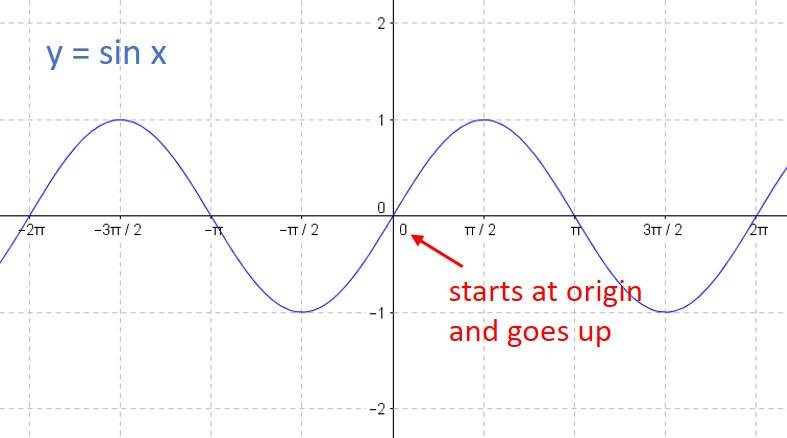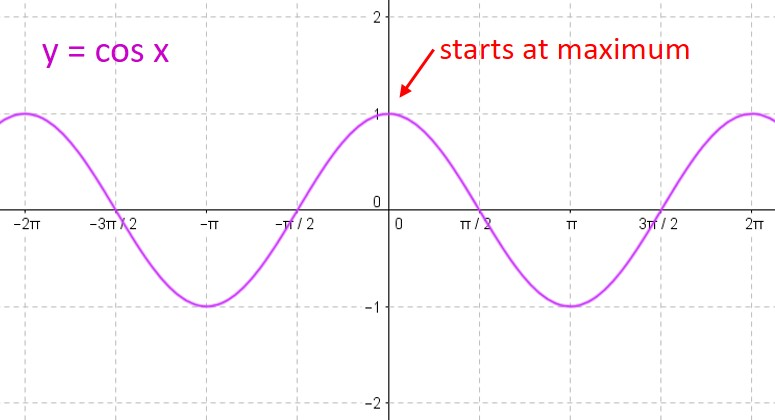2)     For either curve, you can break one period into four equal intervals. At each interval, the curve moves from its midline to the maximum to the midline to the minimum to the midline to the maximum to… over and over again. So all you need to do is find the starting point, and plot the points on the curve at each ¼-period interval.

We will always write our functions in standard form:$f(x) = A \; sin(Bx+C) + D \; or \; f(x)= A \; cos(Bx+C) + D$

(Note that some textbooks prefer to write the formula in a slightly different form:$f(x) = A \; sin(B(x+C)) + D \; or \; f(x)= A \; cos(B(x+C)) + D$

We will discuss how that affects your work below.)

Each of the constants A, B, C & D affects the position of the curve and you need to analyze this before you graph the curve. Let’s look at each of them in turn:

A: The absolute value of this number tells you the amplitude of your curve.

B: The period of your curve is determined by dividing$2\pi$ by B.

C: The phase shift is found by dividing -C by B. A positive value means the phase shift is to the right. A negative value means the phase shift is to the left. (If your class uses the version of the equation above with the B factored out, then the phase shift is equal to C.)

D: The vertical shift is equal to D.

Here’s an example to show how you would calculate all these values.$\displaystyle f(x) = -3 \sin (2x + \frac{\pi}{2}) -1$

Here, A = -3; B = 2; C =$\pi/2$; and D = -1. Therefore,

Amplitude = |-3| = 3

Period =$2 \pi /2 = \pi$

Phase shift =$- (\pi /2)/2 = - \pi /4$ (that is,$\pi /4$ units to the left)

Vertical shift = -1

When you need to graph a sine or cosine curve, always determine these four values first. Then you are ready to graph the function. We’ll do that in our next post.

Blue Taste Theme created by Jabox